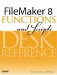# Month()

Category: Date

 Syntax: Month ( date )

Parameters:

dateAny valid date (1/1/000112/31/4000). The parameter should be a string containing a date (for example, "3/17/2004"), an expression with a date result (for example, Date (6, 29, 1969)), or an integer that represents a serialized date value (for example, 718977).

Data type returned: Number

Description:

Returns the month number (112) for any valid date (1/1/000112/31/4000).

The numeric value returned by Month() can be used in mathematical calculations as well as within the Date() function to construct a new date.

One common use of the Month() function is to build a formula that returns the quarter of a given date:

``` Case(
Month(myDate) < 4, "First Quarter",
Month(myDate) < 7, "Second Quarter",
Month(myDate) < 9, "Third Quarter",
"Fourth Quarter"
)
```

Examples:

Function

Results

Month ("5/1/2000")

Returns 5.

Month (718977)

Returns 6.

Month (Get (CurrentDate))

Returns 3 (if the current date is in March).FileMaker 8 Functions and Scripts Desk Reference
ISBN: 0789735113
EAN: 2147483647
Year: 2004
Pages: 352